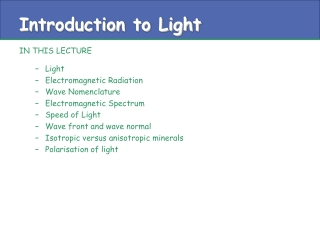Download PresentationIntroduction to Light

Loading in 2 Seconds...

# Introduction to Light

Download Presentation## Introduction to Light

- - - - - - - - - - - - - - - - - - - - - - - - - - - E N D - - - - - - - - - - - - - - - - - - - - - - - - - - -
##### Presentation Transcript

1. Introduction to Light IN THIS LECTURE • Light • Electromagnetic Radiation • Wave Nomenclature • Electromagnetic Spectrum • Speed of Light • Wave front and wave normal • Isotropic versus anisotropic minerals • Polarisation of light

2. Light • LIGHT is a form of energy, detectable with the eye, which can be transmitted from one place to another at finite velocity. • Two complimentary theories have been proposed to explain how light behaves and the form by which it travels. • Particle theory - release of a small amount of energy as a photon when an atom is excited. • Wave theory - radiant energy travels as a wave from one point to another. • Wave theory effectively describes the phenomena of polarization, reflection, refraction and interference, which form the basis for optical mineralogy.

3. Electromagnetic Radiation Wave Theory tells us that radiant energy like light has both electrical and magnetic properties and is therefore called Electromagnetic Radiation Light rays haveelectric and magnetic components that vibrate at right angles to each other and to the direction of propagation. In optical mineralogy only the electric component, referred to as the electric vector, is considered and is referred to as the vibration direction of the light ray

4. Wave Nomenclature • The wave is travelling left to right with velocity (V nm/sec). The wavelength (l, nm) is the distance between successive wave crests. The frequency is the number of wave crests that pass some point per second. Frequency is expressed as cycles per second or Hertz (Hz). The amplitude (A) is the height of the wave. • Velocity, wavelength and frequency are related by the following equation f = V/l

5. Electromagnetic Spectrum The electromagnetic Spectrum classifies different types of energy in terms of wavelength. Energy in the form of visible light, with wavelengths between about 400nm and 700nm, is a small portion of this spectrum (1nm = 10-9m). Note that the energy of light increases with decreasing wavelength

6. Speed of Light • The velocity of light depends on the nature of the material through which it travels and the wavelength of the light • In a vacuum the velocity of light is at a maximum: 3 x 1017 nm/sec In all other material, the velocity of light slows down. This is because the wavelength of light decreases as the density of the material through which it is travelling increases but the frequency remains constant.

7. Wave front & Wave Normal A wave front is a surface that connects the same points on adjacent waves Successive wave fronts are one wavelength apart A wave normal is right angles to the wave front and represents the direction the wave is moving in A light ray is the propagation direction of the light energy

8. Isotropic versus Anisotropic Minerals can be subdivided, based on the interaction of the light ray travelling through the mineral and the nature of the chemical bonds holding the mineral together, into two classes: ISOTROPIC ANISOTROPIC • Same velocity of light in all directions • Wave Normal and Light Ray are parallel • Examples are volcanic glass and minerals of the isometric (cubic) system such as Fluorite, Garnet and Halite • Velocity of light is different in different directions • Wave Normal and Light Ray are NOT parallel • Anisotropic minerals belong to tetragonal, hexagonal, orthorhombic, monoclinic and triclinic systems

9. Isotropic vs Anisotropic ISOTROPIC In isotropic minerals the wave normal and the direction of propagation of the light rays are perpendicular to the wave front ANISOTROPIC In anisotropic minerals the light rays are not perpendicular to the wave front

10. Polarisation of Light • Light emanating from some source, sun, or a light bulb, vibrates in all directions at right angles to the direction of propagation and is unpolarized. • If the electric vector is constrained to lie within one plane then the light is plane-polarised and vibrates in only one direction.